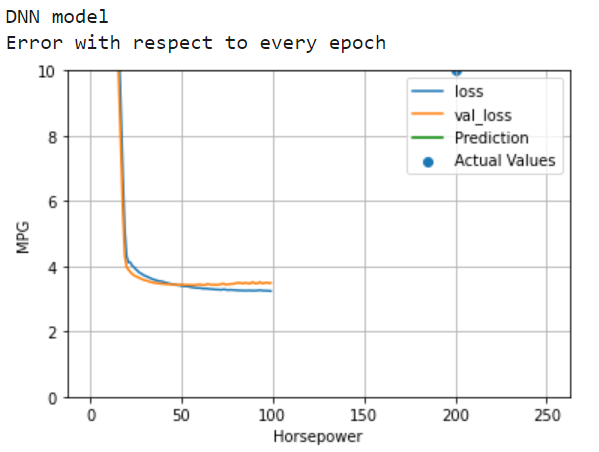# How can a DNN (deep neural network) model be built on Auto MPG dataset using TensorFlow?

PythonServer Side ProgrammingProgrammingTensorflow

#### Beyond Basic Programming - Intermediate Python

Most Popular

36 Lectures 3 hours

#### Practical Machine Learning using Python

Best Seller

91 Lectures 23.5 hours

#### Practical Data Science using Python

22 Lectures 6 hours

Tensorflow is a machine learning framework that is provided by Google. It is an open−source framework used in conjunction with Python to implement algorithms, deep learning applications and much more. It is used in research and for production purposes.

Tensor is a data structure used in TensorFlow. It helps connect edges in a flow diagram. This flow diagram is known as the ‘Data flow graph’. Tensors are nothing but multidimensional array or a list.

The dataset we use is called the ‘Auto MPG’ dataset. It contains fuel efficiency of 1970s and 1980s automobiles. It includes attributes like weight, horsepower, displacement, and so on. With this, we need to predict the fuel efficiency of specific vehicles.

We are using the Google Colaboratory to run the below code. Google Colab or Colaboratory helps run Python code over the browser and requires zero configuration and free access to GPUs (Graphical Processing Units). Colaboratory has been built on top of Jupyter Notebook. Following is the code snippet −

## Example

print("DNN model")
history = dnn_horsepower_model.fit(
train_features['Horsepower'], train_labels,
validation_split=0.2,
verbose=0, epochs=100)
print("Error with respect to every epoch")
plot_loss(history)
x = tf.linspace(0.0, 250, 251)
y = dnn_horsepower_model.predict(x)
plot_horsepower(x, y)
test_results['dnn_horsepower_model'] = dnn_horsepower_model.evaluate(
test_features['Horsepower'], test_labels,
verbose=0)

## Output## Explanation

• DNN refers to a deep neural network, and in this case it has a single input, i.e the ‘Horsepower’.

• This model is fit to the training data.

• The statistical parameters stored in ‘history’ is plotted on the console.

• The predictions are made and these are evaluated using the ‘evaluate’ method.